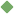physics 1. [ noun ] (physics) the science of matter and energy and their interactions Synonyms: physical_science natural_philosophy Related terms: natural_science electronics mechanics astronomy crystallography thermodynamics nuclear_physics optics rheology quantum_physics cryogenics electrostatics biophysics aeronautics solid-state_physics electromagnetism statistical_mechanics plasma_physics acoustics particle_physics wave_theory relativity kinetic_theory quantum_theory corpuscular_theory power light energy shear force atom tension molecule work wave diffusion amplitude collision strain critical nuclear free absorption bound disintegrate physicist gravity polarize magnet freeze magnetize viscoelastic neutral relativistic aerodynamic gravitational_theory oscillation crystallize hyperfine acceleration chaos recombination isotropy antinode Dalton's_law duality flux_density Gay-Lussac's_law law_of_gravitation identical metastability aeolotropic electrify demagnetize inertia sympathetic_vibration induce reluctance transform interaction weak_interaction reactive weakly_interacting_massive_particle nuclear_reaction activated transform liquefy repulsive modulus unreactive dip absorber G disintegrate atomic_spectrum perturbation gas_constant conservation Bohr_theory decouple liquefy parity wave_theory fiducial corpuscular_theory metastable depolarise kinetic_theory dynamic relativity hydrodynamic miscible solidify backscatter reversibly supersymmetry quantum_theory rheologic mesonic mass_energy quantal quantise immiscible bose-einstein_statistics quantum fermi-dirac_statistics boltzmann_distribution_law quantum_jump containment node kirchhoff's_laws solitary_wave transmutation wave_front hooke's_law cohesion coriolis_force law_of_thermodynamics planck's_law nuclear_reactor hodoscope diabatic solidify planck's_radiation_law principle_of_relativity magnetic_dipole_moment principle_of_equivalence inductance absorptivity gravity_wave rest_mass strong_interaction reluctivity center_of_buoyancy adiabatic coriolis_effect transient relaxation attracter gravitational_mass relativistic_mass coefficient_of_elasticity mass-energy_equivalence inertial_mass field_theory dynamical_system stress nonfissionable fissionable scintillation quench attractive abundance spallation metric pencil ground_state chaotic phase_space To share this definition press "text" (Facebook, Twitter) or "link" (blog, mail) then paste text link Similar spelling: physic   physique